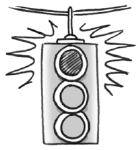### Home > CCA2 > Chapter 7 > Lesson 7.2.3 > Problem7-148

7-148.George was solving the equation $\left(2x − 1\right)\left(x + 3\right) = 4$ and he got the solutions $x =\frac { 1 } { 2 }$ and $x = −3$. Jeffrey came along and said, “You made a big mistake! You set each factor equal to zero, but it's not equal to zero, it's equal to $4$. So you have to set each factor equal to 4 and then solve.” Who is correct? Show George and Jeffrey how to solve this equation. To be sure that you are correct, check your solutions.

To use the Zero Product Property, you need a product equal to zero.

Multiply the binomials, subtract $4$ to make the equation equal to zero, and factor the resulting trinomial.
Then use the Zero Product Property.